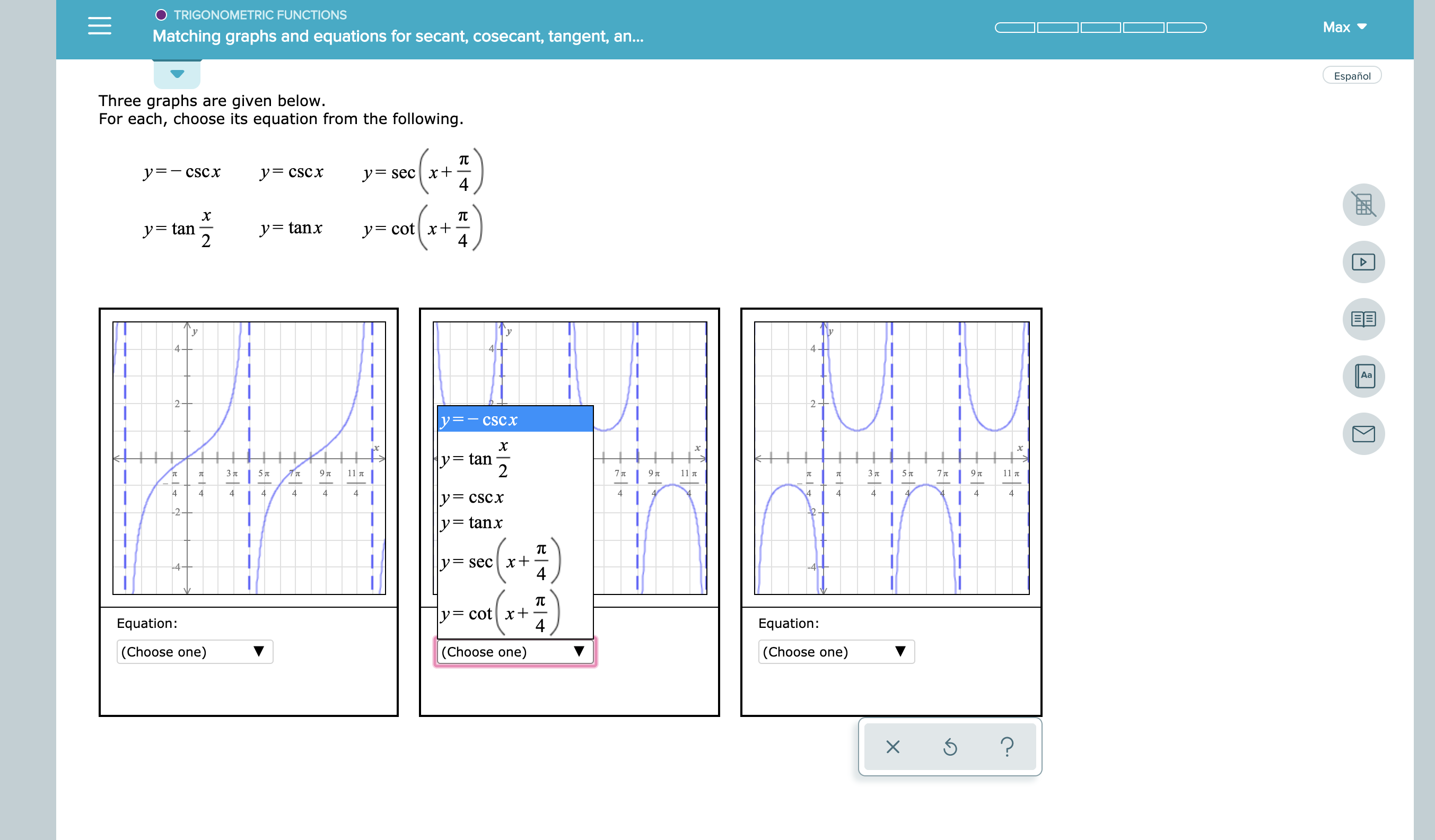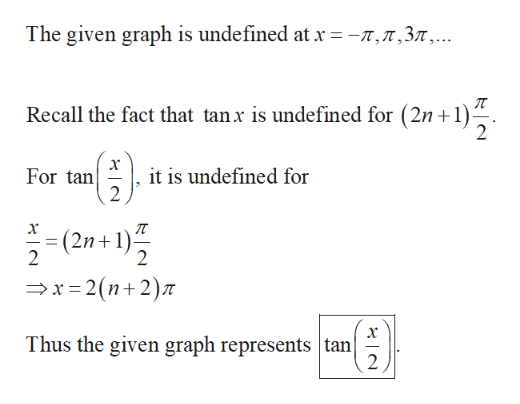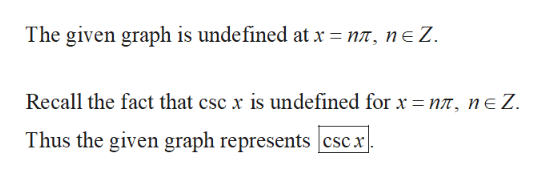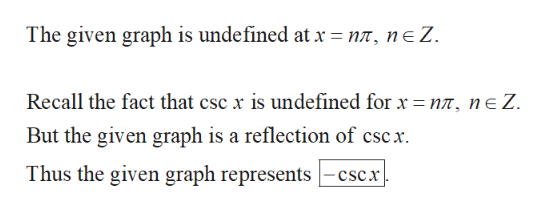# O TRIGONOMETRIC FUNCTIONSMaxMatching graphs and equations for secant, cosecant, tangent, an...EspañolThree graphs are given below.For each, choose its equation from the following.тy secx+4y CSCXyCSCxTCXycotx+4ytan2y=tanx44Aa22y-csCxXy= tan23 75 797T11 7T7 T97T11 T3 7T577 79 71144444444444444yCSCx2y= tanxтх+4ysecтycot x+4Equation:Equation:(Choose one)(Choose one)(Choose one)?

Question
1 views

See attachmenthelp_outlineImage TranscriptioncloseO TRIGONOMETRIC FUNCTIONS Max Matching graphs and equations for secant, cosecant, tangent, an... Español Three graphs are given below. For each, choose its equation from the following. т y secx+ 4 y CSCX yCSCx TC X ycotx+ 4 ytan 2 y=tanx 4 4 Aa 2 2 y-csCx X y= tan 2 3 7 5 7 97T 11 7T 7 T 97T 11 T 3 7T 57 7 7 9 7 11 4 4 4 4 4 4 4 4 4 4 4 4 4 4 yCSCx 2 y= tanx т х+ 4 ysec т ycot x+ 4 Equation: Equation: (Choose one) (Choose one) (Choose one) ? fullscreen
check_circle

Step 1

Observe the first graph.help_outlineImage TranscriptioncloseThe given graph is undefined at x = -n,n,37,.. Recall the fact that tanx is undefined for (2n+1) х it is undefined for 2 For tan - (2n+1) 2 2 x 2(n2)7 Thus the given graph represents tan 2 fullscreen
Step 2

Observe the third graph.help_outlineImage TranscriptioncloseThe given graph is undefined at x = nn, ne Z Recall the fact that csc x is undefined for x = nn, ne Z Thus the given graph represents csCx fullscreen
Step 3

Observe the seco...help_outlineImage TranscriptioncloseThe given graph is undefined at x = nn, ne Z Recall the fact that csc x is undefined for x n, ne Z. But the given graph is a reflection of cscx Thus the given graph representscscx fullscreen

### Want to see the full answer?

See Solution

#### Want to see this answer and more?

Solutions are written by subject experts who are available 24/7. Questions are typically answered within 1 hour.*

See Solution
*Response times may vary by subject and question.
Tagged in

### Other